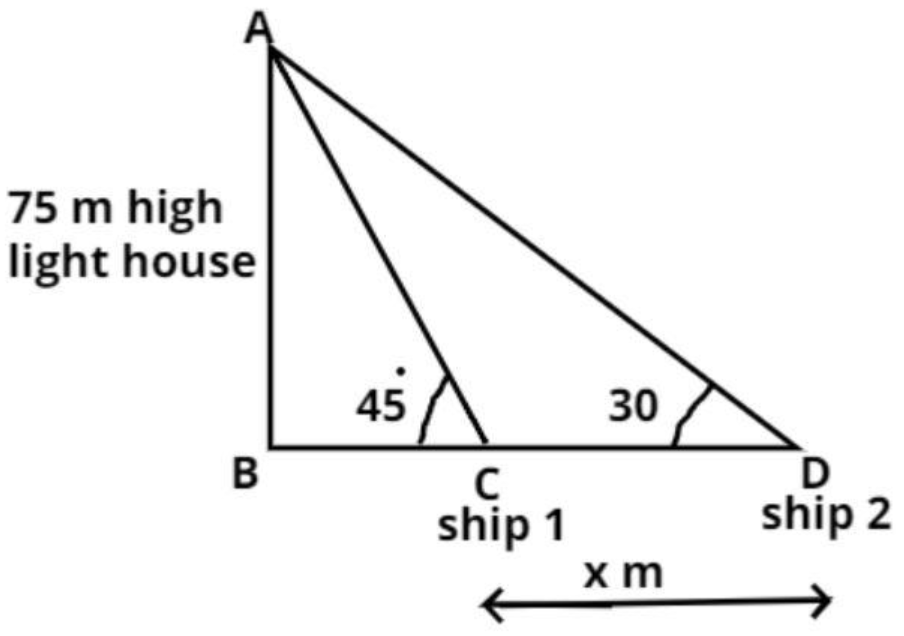QuestionAnswers

# As observed from the top of a 75m high lighthouse from the sea-level, the angles of depression of two ships are 30 degree and 45 degree. If one ship is exactly behind the other on the same side of the lighthouse, find the distance between the two ships.Verified
54.2k+ views
Hint: This is a standard problem involving the concept of height and distances. Simple diagrammatic representation based upon the information provided will help in getting a clearer picture about the triangles that will be used to solve this problem. Then use of the basic trigonometric ratios when applied to specific triangles will help in getting the answer.Let AB be the light house. The height (h) of the lighthouse from the sea level is AB = h = 75 m.
Now the angle of depression of the ships is 30 degree and 45 degree and the one ship is exactly behind the other one on the same side of the light house (see figure).
Now we have to find out the distance between the ships.
Let the distance be x m (see figure).
$\Rightarrow CD = x$ m
In triangle ABC,
$\tan {45^0} = \dfrac{{{\text{Perpendicular}}}}{{{\text{Base}}}} = \dfrac{{AB}}{{BC}} = \dfrac{{75}}{{BC}}$
As we know $\tan {45^0} = 1$
$\Rightarrow BC = 75$m
Now in triangle ABD
$\tan {30^0} = \dfrac{{{\text{Perpendicular}}}}{{{\text{Base}}}} = \dfrac{{AB}}{{BD}}$
As we know $\tan {30^0} = \dfrac{1}{{\sqrt 3 }}$
So substitute this value in above equation we have,
$\Rightarrow \dfrac{1}{{\sqrt 3 }} = \dfrac{{75}}{{BD}}$
$\Rightarrow BD = 75\sqrt 3$ m
So from figure CD = BD – BC
So, substitute the values of BD and BC in above equation we have
$\therefore CD = 75\sqrt 3 - 75$
$\Rightarrow x = 75\left( {\sqrt 3 - 1} \right)$ m
So, the distance between two ships is $75\left( {\sqrt 3 - 1} \right)$ meter.
So, this is the required answer.

Note: Whenever we face such height and distances problems the key point is about the basic understanding of the simple trigonometric ratios and their formulas like that is sin, cos and tan. The application of these trigonometric ratios will set you on the right track to get the required answer.